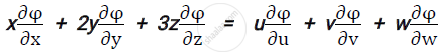# Applied Mathematics 1 CBCGS 2016-2017 BE Civil Engineering Semester 1 (FE First Year) Question Paper Solution

Applied Mathematics 1 [CBCGS]
Date: December 2016

(1) Question no. 1 is compulsory.
(2) Attempt any 3 questions from remaining five questions.

1
1.a

If cos alpha cos beta=x/2, sinalpha sinbeta=y/2, prove that:

sec(alpha -ibeta)+sec(alpha-ibeta)=(4x)/(x^2-y^2)

Concept: Review of Complex Numbers‐Algebra of Complex Number
Chapter:  Complex Numbers
1.b

If z =log(e^x+e^y) "show that rt" - s^2 = 0  "where r"= (del^2z)/(delx^2),t=(del^2z)/(dely^2)"s"=(del^2z)/(delx dely)

Concept: Review of Complex Numbers‐Algebra of Complex Number
Chapter:  Complex Numbers
1.c

If x = uv, y =(u+v)/(u-v).find (del(u,v))/(del(x,y)).

Concept: Review of Complex Numbers‐Algebra of Complex Number
Chapter:  Complex Numbers
1.d

If y=2^xsin^2x cosx find y_n

Concept: Review of Complex Numbers‐Algebra of Complex Number
Chapter:  Complex Numbers
1.e

Express the matrix as the sum of symmetric and skew symmetric matrices.

Chapter:  Matrices
1.f

Evaluat lim_(x->0) (e^(2x)-(1+x)^2)/(xlog(1+x)

Concept: L‐ Hospital Rule
Chapter:  Indeterminate Forms, Numerical Solutions of Transcendental Equations and System of Linear Equations
2
2.a

Show that the roots of x5 =1 can be written as 1, alpha^1,alpha^2,alpha^3,alpha^4 .hence show that (1-alpha^1) (1-alpha^2) (1-alpha^3)(1-alpha^4)=5.

Concept: Powers and Roots of Trigonometric Functions
Chapter:  Complex Numbers
2.b

Reduce the following matrix to its normal form and hence find its rank.

Concept: Reduction to Normal Form
Chapter:  Matrices
2.c

Solve the following equation by Gauss-Seidel method upto four iterations

4x-2y-z=40, x-6y+2y=-28, x-2y+12z=-86.

Concept: Gauss Seidal Iteration Method
Chapter:  Indeterminate Forms, Numerical Solutions of Transcendental Equations and System of Linear Equations
3
3.a

Investigate for what values of μ and λ the equations x+y+z=6, x+2y+3z=10, x+2y+λz=μ has
1) No solution
2) A unique solution
3) Infinite number of solutions.

Concept: consistency and solutions of homogeneous and non – homogeneous equations
Chapter:  Matrices
3.b

If u=x^2+y^2+z^2 where x=e^t, y=e^tsint,z=e^tcost

Prove that (du)/(dt)=4e^(2t)

Concept: Review of Complex Numbers‐Algebra of Complex Number
Chapter:  Complex Numbers
3.c

Show that sin(e^x-1)=x^1+x^2/2-(5x^4)/24+...................

Concept: Expansion of 𝑒^𝑥 , sin(x), cos(x), tan(x), sinh(x), cosh(x), tanh(x), log(1+x), 𝑠𝑖𝑛−1 (𝑥),𝑐𝑜𝑠−1 (𝑥),𝑡𝑎𝑛−1 (𝑥)
Chapter:  Applications of Partial Differentiation , Expansion of Functions
3.d

Expand 2x^3+7x^2+x-6 in powers of (x-2)

Concept: Expansion of sinnθ, cosnθ in powers of sinθ, cosθ
Chapter:  Complex Numbers
4
4.a

If x = u+v+w, y = uv+vw+uw, z = uvw and φ is a function of x, y and z
Prove thatConcept: Euler’s Theorem on Homogeneous functions with two and three independent variables (with proof)
Chapter:  Partial Differentiation
4.b

If tan(θ+iφ)=tanα+isecα
Prove that
1)e^(2varphi)=cot(varphi/2)
2) 2theta=npi+pi/2+alpha

Concept: Euler’s Theorem on Homogeneous functions with two and three independent variables (with proof)
Chapter:  Partial Differentiation
4.c

Find the roots of the equation x^4+x^3 -7x^2-x+5 = 0 which lies between 2 and 2.1 correct to 3 places of decimals using Regula Falsi method.

Concept: Regula – Falsi Equation
Chapter:  Indeterminate Forms, Numerical Solutions of Transcendental Equations and System of Linear Equations
5
5.a

If y=(x+√x2-1 ,Prove that

(x^2-1)y_(n+2)+(2n+1)xy_(n+1)+(n^2-m^2)y_n=0

Concept: Leibnitz’S Theorem (Without Proof) and Problems
Chapter: [6.01] Successive Differentiation
5.b

Using the encoding matrix [(1,1),(0,1)] encode and decode the messag I*LOVE*MUMBAI.

Concept: Application of Inverse of a Matrix to Coding Theory
Chapter:  Matrices
5.c

Considering only principal values separate into real and imaginary parts

i^((log)(i+1))

Concept: Separation of Real and Imaginary Parts of Logarithmic Functions
Chapter: [6.02] Logarithm of Complex Numbers
5.d

Show that ilog((x-i)/(x+i))=pi-2tan6-1x

Concept: Logarithmic Functions
Chapter: [6.02] Logarithm of Complex Numbers
6
6.a

Using De Moivre’s theorem prove that]

cos^6theta-sin^6theta=1/16(cos6theta+15cos2theta)

Concept: D’Moivre’S Theorem
Chapter:  Complex Numbers
6.b

If u =sin^(-1)((x^(1/3)+y^(1/3))/(x^(1/2)-y^(1/2))), Prove that

x^2(del^2u)/(delx^2)+2xy(del^2u)/(delxdely)+y^2(del^2u)/(dely^2)=tanu/144(tan^2u+13)

Concept: System of Homogeneous and Non – Homogeneous Equations
Chapter:  Matrices
6.c

Find the maxima and minima of x^3 y^2(1-x-y)

Concept: Maxima and Minima of a Function of Two Independent Variables
Chapter:  Applications of Partial Differentiation , Expansion of Functions

#### Request Question Paper

If you dont find a question paper, kindly write to us

View All Requests

#### Submit Question Paper

Help us maintain new question papers on Shaalaa.com, so we can continue to help students

only jpg, png and pdf files

## University of Mumbai previous year question papers Semester 1 (FE First Year) Applied Mathematics 1 with solutions 2016 - 2017

University of Mumbai Semester 1 (FE First Year) Applied Mathematics 1 question paper solution is key to score more marks in final exams. Students who have used our past year paper solution have significantly improved in speed and boosted their confidence to solve any question in the examination. Our University of Mumbai Semester 1 (FE First Year) Applied Mathematics 1 question paper 2017 serve as a catalyst to prepare for your Applied Mathematics 1 board examination.
Previous year Question paper for University of Mumbai Semester 1 (FE First Year) Applied Mathematics 1-2017 is solved by experts. Solved question papers gives you the chance to check yourself after your mock test.
By referring the question paper Solutions for Applied Mathematics 1, you can scale your preparation level and work on your weak areas. It will also help the candidates in developing the time-management skills. Practice makes perfect, and there is no better way to practice than to attempt previous year question paper solutions of University of Mumbai Semester 1 (FE First Year).

How University of Mumbai Semester 1 (FE First Year) Question Paper solutions Help Students ?
• Question paper solutions for Applied Mathematics 1 will helps students to prepare for exam.
• Question paper with answer will boost students confidence in exam time and also give you an idea About the important questions and topics to be prepared for the board exam.
• For finding solution of question papers no need to refer so multiple sources like textbook or guides.
Share